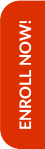Olympiads are the stepping stones to achieve better results in the competitive world that lies ahead in the life of the child. Math Olympiad examinations help students to improve their mathematical skills along with their analytical and problem solving abilities.

Hence, Olympiad Success Live has designed the course for Math Olympiad for class 2 in such a way that the foundation of the child is built up. For this, we have done great efforts in finding the tutor for class 2 Math Olympiad with relevant background and experience.

If you are interested in purchasing this course, then please Enrol Now. You will be redirected to the batch detail page, wherein you can see all the details like batch start and demo dates, fess and the registration link related to Math Olympiad for class 2 course.

##### Syllabus

Numerals, Number Names and Number Sense

• Even and odd numbers
• Numbers and number names
• Counting – Breaks
• Before – After – Between
• 3-digit numbers on Abacus
• Face value and Place value
• Expanded Form and Short form
• Comparison of Numbers
• Ascending and Descending orders

Computation Operations

• Subtraction: Subtraction up to 3-digit numbers (without and with carrying) and word problems on subtraction
• Multiplication: Multiplication up to 3-digit numbers by a one-digit number and word problems on multiplication
• Division: Making groups, division as the inverse of multiplication and word problem on division

Measurement of Length

• Units of length
• Measure the length of an object using a ruler
• Addition and Subtraction of Lengths
• Word problem

Measurement of Weight

• Units of weight
• Measuring Weights
• Comparison of weights
• Addition and Subtraction of Weights
• Word Problems

Measurement of Capacity

• Comparison of containers and making orders according to their volume
• Addition and Subtraction of Capacities
• Word Problems

Time and Temperature

• Clocks
• Daily Routine
• Days of the week
• Today, yesterday and tomorrow
• Months of a Year
• Calendar
• Choose temperature on the thermometer

Money

• Identifying currencies, notes and coins
• Addition and Subtraction of Money
• Word problems

Geometry

• Point and Lines
• Horizontal, Vertical, and Slanting lines
• Straight and Curved lines
• Plane figures and solids
• Identifying 2-D shapes such as rectangle, square, triangle and circles
• Identifying 3-D shapes such as cube, cuboid, cylinder, cone and sphere

Pictograph

• Introduction to Pictograph
• Questions based on pictures

Pattern

• Number Pattern
• Analysing Pattern
• Geometrical Pattern
• What comes next

Online classes started for English, Mathematics, Science and Reasoning for classes (3rd to 10th)